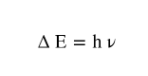# Problem: Ionization involves completely removing an electron from an atom. Light of a particular wavelength can cause ionization to occur if it has the required energy. The energy to ionize a certain element is 530 kJ/mol. What wavelength contains enough energy in a single photon to ionize one atom of this element? Enter your answer with three significant figures.

###### FREE Expert Solution
87% (405 ratings)
###### FREE Expert Solution
• The equation for energy is:• Wavelength (λ) is related to frequency (v) by the equation:

87% (405 ratings)###### Problem Details

Ionization involves completely removing an electron from an atom. Light of a particular wavelength can cause ionization to occur if it has the required energy. The energy to ionize a certain element is 530 kJ/mol. What wavelength contains enough energy in a single photon to ionize one atom of this element? Enter your answer with three significant figures.

What scientific concept do you need to know in order to solve this problem?

Our tutors have indicated that to solve this problem you will need to apply the Wavelength and Frequency concept. You can view video lessons to learn Wavelength and Frequency. Or if you need more Wavelength and Frequency practice, you can also practice Wavelength and Frequency practice problems.

What is the difficulty of this problem?

Our tutors rated the difficulty ofIonization involves completely removing an electron from an ...as medium difficulty.

How long does this problem take to solve?

Our expert Chemistry tutor, Sabrina took 5 minutes undefined to solve this problem. You can follow their steps in the video explanation above.

What professor is this problem relevant for?

Based on our data, we think this problem is relevant for Professor Sumner's class at UF.